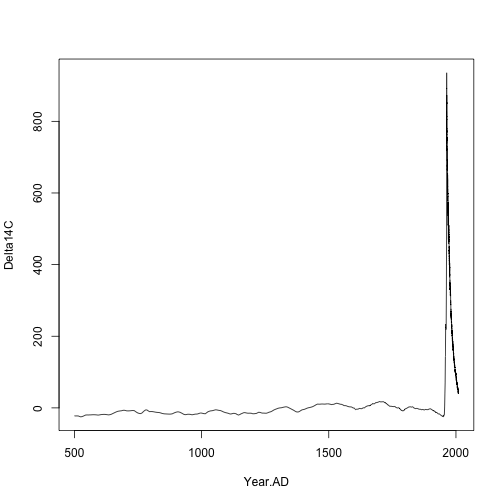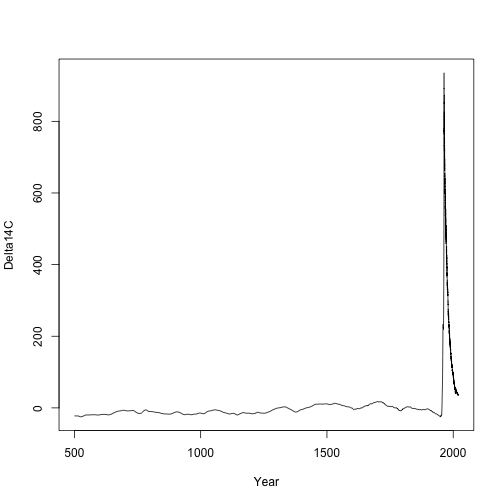In radiocarbon applications, it is often necessary to bind atmospheric radiocarbon curves from different sources. Here, I show an example on how to bind a pre- and post-bomb curve as well as a forecasted curve for which atmospheric radiocarbon data have not been released yet.

As an example, we will consider the case in which we need an atmospheric radiocarbon curve from the years 500 to 2020 AD. This implies binding first the IntCal09 curve with one of the post-bomb curves, and then doing a forecast from the year 2009 to 2020.

## Binding pre- and post-bomb curves

SoilR includes already two prebomb datasets: IntCal09 and IntCal13, and four curves for the post-bomb period stored as a list in the object Hua2013. SoilR also includes a handy function called bind.C14curves that allows you to bind a pre- and a post-bomb curves. This function has three arguments: prebomb, postbomb, and time.scale. The prebomb argument accepts either IntCal09 or IntCal13, and we recommend to use IntCal13 because it is the curve currently recognized as official by the radiocarbon community. The postbomb argument requires the specification of the hemispheric zone as described in Hua et al. (2013). The time.scale argument determines whether the results are reported in a time scale in years before present (BP) or anno domini (AD).

In this example, we will bind the IntCal13 and the post-bomb curve for the northern hemisphere zone 2, which corresponds to most of the temperate zone in this hemisphere.

preandpost=bind.C14curves(prebomb=IntCal13,postbomb=Hua2013$NHZone2,time.scale="AD")  The combined dataset goes from 50,000 years before present (-500000 AD) to the year 2009.62. The dataset is therefore very large: dim(preandpost)  ##  5694 3  We are only interested in values of Delta14C starting from the year 500, so we select only the values of interest and plot the results bombcurve=preandpost[preandpost[,1]>=500,1:2] plot(bombcurve[,1:2],type="l")## Forecast of the bomb curve We use the R package forecast to predict the values of atmospheric radiocarbon until the year 2020. library(forecast)  The forecast algorith requires equally spaced values for the radiocarbon time series, however the original time series is not equally spaced, for this reason we need to create a new series based on an interpolation procedure using a spline function yrs=seq(1966,2009.5,by=1/4) # A series of years by quarters nz2=spline(Hua2013$NHZone2[,c(1,4)],xout=yrs) #Spline interpolation of the NH_Zone 2 dataset at a quaterly basis
nhz2=ts((nz2$y-1)*1000,start=1966,freq=4) #Transformation into a time-series object  Now, we use the ets function to fit an exponential smoothing state space model, and then perform a forecast based on this model m=ets(nhz2) #Fits an exponential smoothing state space model to the time series f2=forecast(m,h=11*4) #Uses the fitted model to forecast 11 years into the future  Now we are ready to combine both curves bc=data.frame(Year=c(bombcurve[-dim(bombcurve),1], seq(tsp(f2$mean),tsp(f2$mean), by=1/tsp(f2$mean))),
Delta14C=c(bombcurve[-dim(bombcurve),2],as.numeric(f2\$mean)))


This data.frame is now ready to use in SoilR. We can now plot this final dataset.

plot(bc,type="l")